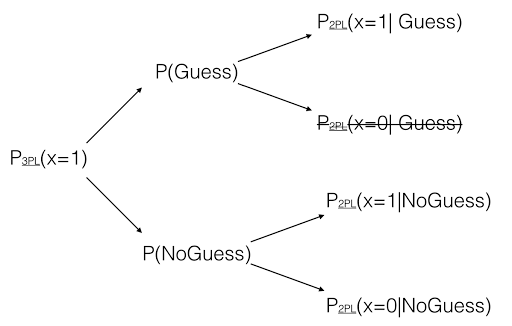[This article was first published on Data Literacy - The blog of Andrés Gutiérrez, and kindly contributed to R-bloggers]. (You can report issue about the content on this page here)
Want to share your content on R-bloggers? click here if you have a blog, or here if you don't.

As I currently am the NPM for PISA in Colombia, I must assist to several meetings dealing with the proper implementation of this assessment in my country. Few of them are devoted to the analysis of this kind of data (coming from IRT models). As usual, OECD has hired organizations with high technical standards. The institute that handles all this data and take part in the analysis is ETS (Educational Testing Services).

PISA and ETS are changing from Rasch models to 2PL models. That involves a significant technical effort to maintain comparability along time. I had the opportunity to talk with some experts from ETS last year, and I formulated them the following question: ¿why to consider 2PL models instead of 3PL models? Well, the answer was not easy, and I am not pretending to explain it here in detail, in part because I am still convinced about the advantages of 3PL models that exceed those of 2PL models. However, they yielded me to a recent paper entitled Is There Need for the 3PL Model? Guess What?

The article was written by Mathias von Davier from ETS. I liked the way von Davier showed 3PL models, as coming from a total probability setup involving 2PL models. Consider the following hierarchical structure: first, the test-taker decides whether he/she is answering that item by guessing or not; then, he\she uses his\her ability to found the correct response. So, the stochastic process behind this structure can be easily shown in a tree diagram:Remember that 3PL models can be written as:

$$P_{3PL}(x=1) = P(Guess) + P(NoGuess) \times P_{2PL}(x=1|NoGuess)$$

Note that, per the model, once the student has chosen to answer by guessing, a correct answer is always found (kind of weird, isn’t it?). So, a major criticism against 3PL models is related to this last point. In R, you can estimate 3PL models by using the mirt package. So, for example, when using the LSAT7 data on the second item, we can estimate this guessing parameter.

library(mirt)
data <- expand.table(LSAT7)
md2  <-  mirt(data, 1,  = '3PL', IRTpars = TRUE)
coef(md2, IRTpars = TRUE)\$Item.2


We found that the guessing parameter is estimated as 0.295. This way, the model is specified as:

$$P_{3PL}(x=1) = 0.295 + 0.705 \times P_{2PL}(x=1|NoGuess)$$